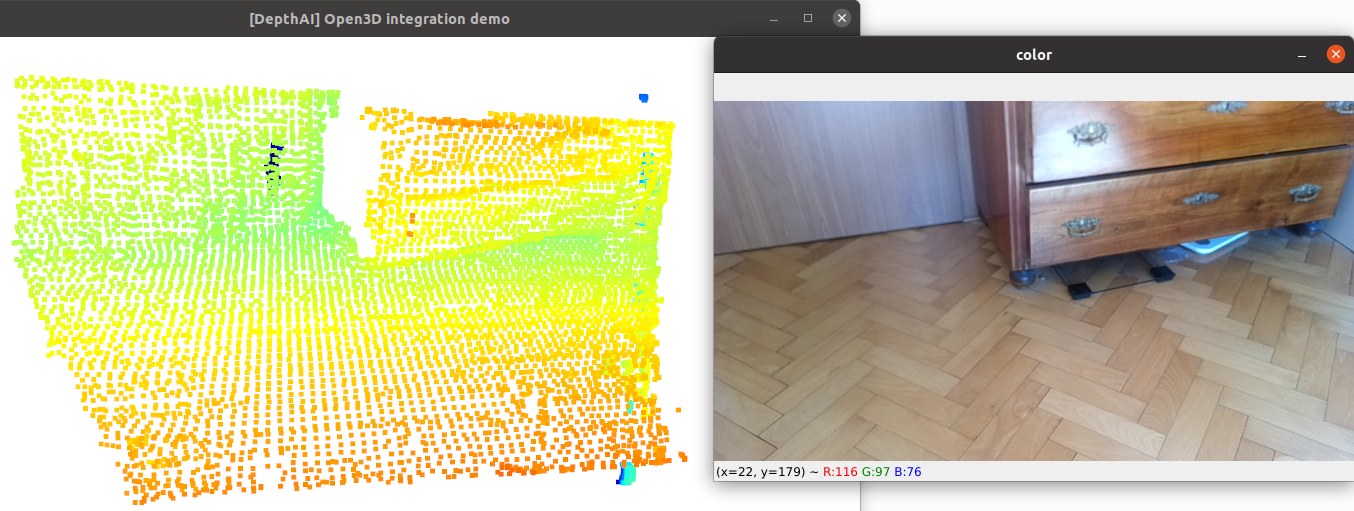# On-device Pointcloud NN model¶

At the time of writing, DepthAI firmware (2.15) doesn’t support converting depth to pointcloud. On the On-device programming page it’s mentioned that Script node shouldn’t be used for any kind of heavy computing, so to convert depth to pointcloud, we would need to create a custom NN model.

Kornia library has a function called depth_to_3d which does exactly that; it returns pointcloud from depth map and camera matrix. A smart person from our Discord community called jjd9 created a working demo of the depth_to_3d logic running on the OAK camera. For C++ version, see code here.## Depth to NN model¶

StereoDepth’s `depth` output is U16 (Unsigned INT 16) datatype. Myriad X only supports FP16 datatype, but with OpenVINO’s Compile Tool you can add conversion layer at the input with `-ip` or `-iop` arguments. These arguments only support FP16 (so no conversion) or U8 (adds U8 -> FP16 layer before the input).

Current workaround is to set conversion for U8 -> FP16 (`-ip U8`). This means the frame will be twice as wide, as each depth pixel will be represented by two (U8) integers. So instead of 640x400 depth frame, the model expects 1280x400 frame.

```# convert the uint8 representation of the image to uint16 (this is needed because the
# converter only allows U8 and FP16 input types)
depth = 256.0 * image[:,:,:,1::2] + image[:,:,:,::2]
```

The code above was added to the model definition. It takes two FP16 numbers and reconstructs the original depth value, so the `depth` tensor has shape 640x400 and is FP16 datatype. This logic can also be seen in the model architecture:## Optimizing the Pointcloud model¶

A few improvements could be made, as:

• The camera matrix is hard-coded into the NN model. This means users would have to create their own NN models, which adds unnecessary package dependencies (pytorch, onnx, onnxsim, blobconverter).

• It’s fairly slow - pointcloud calculation (without visualization) runs at ~19FPS for 640x400 depth frames.

Since the camera matrix (intrinsics) is static, the part below in red could be calculated once instead of being calculated every single depth frame. This should reduce the complexity of the model and improve FPS.Since Kornia library is open source, we can start with the architecture of the depth_to_3d function (code here) and remove the unnecessary part of the model. After moving all the logic to the same function, we end with this code.

We can remove the part of the model that calculates `xyz` vector and calculate it once on the host, then send it to the model and reuse it for every inference. I have also converted the code so it uses numpy functions instead of pytorch ones, to avoid pytorch dependency:

```def create_xyz(width, height, camera_matrix):
xs = np.linspace(0, width - 1, width, dtype=np.float32)
ys = np.linspace(0, height - 1, height, dtype=np.float32)

# generate grid by stacking coordinates
base_grid = np.stack(np.meshgrid(xs, ys)) # WxHx2
points_2d = base_grid.transpose(1, 2, 0) # 1xHxWx2

# unpack coordinates
u_coord: np.array = points_2d[..., 0]
v_coord: np.array = points_2d[..., 1]

# unpack intrinsics
fx: np.array = camera_matrix[0, 0]
fy: np.array = camera_matrix[1, 1]
cx: np.array = camera_matrix[0, 2]
cy: np.array = camera_matrix[1, 2]

# projective
x_coord: np.array = (u_coord - cx) / fx
y_coord: np.array = (v_coord - cy) / fy

xyz = np.stack([x_coord, y_coord], axis=-1)
```

Moving this logic to the host side has significantly reduced the model’s complexity, as seen below.## On-device Pointcloud demo¶

Altering the model has improved the performance of it, but not as much as I would have expected. The demo now runs at about 24FPS (previously 19FPS) for the 640x400 depth frames.

This demo can be found at depthai-experiments.On the host side we only downsample (for faster visualization). For “cleaner” pointcloud we could also remove statistical outliers, but that’s outside the scope of this tutorial.

## Got questions?

Head over to Discussion Forum for technical support or any other questions you might have.